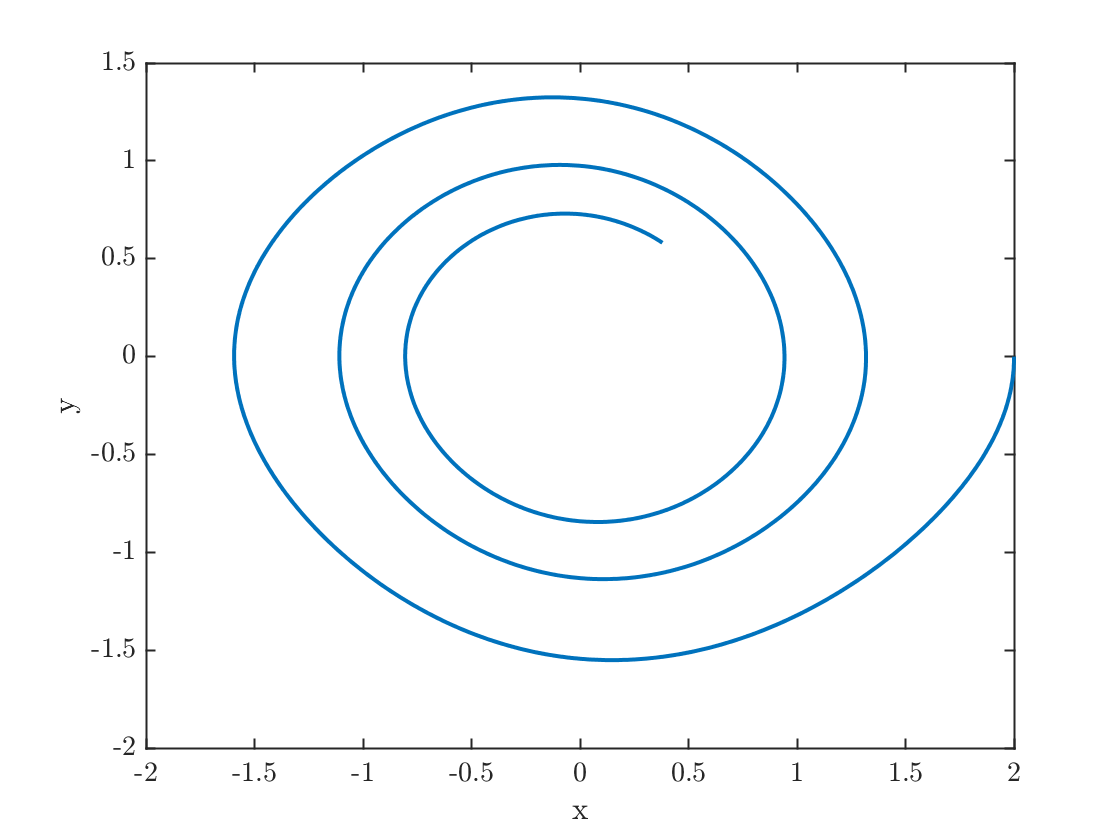# Example of the Euler Method in 2D

We will use the Euler method to solve the damped pendulum equation written as a systemfor initial conditions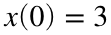,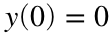, and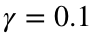.
% Set up initial conditions and parameters
x0=2;
y0=0;
gamma=0.1;
% Set up time stepping
h = 1/100;
tfinal=20;
nsteps=tfinal/h;
t=linspace(0,tfinal,nsteps+1);
% Initialize x & y
x=zeros(size(t));
y = zeros(size(t));
x(1)=x0; y(1)=y0;
% Loop through and run the Euler method
for k=1:nsteps
x(k+1) = x(k) + h * y(k);
y(k+1) = y(k) + h * (-gamma*y(k)-sin(x(k)));
end
% plot the results
plot(t,x,t,y);
xlabel('t'); legend('x','y')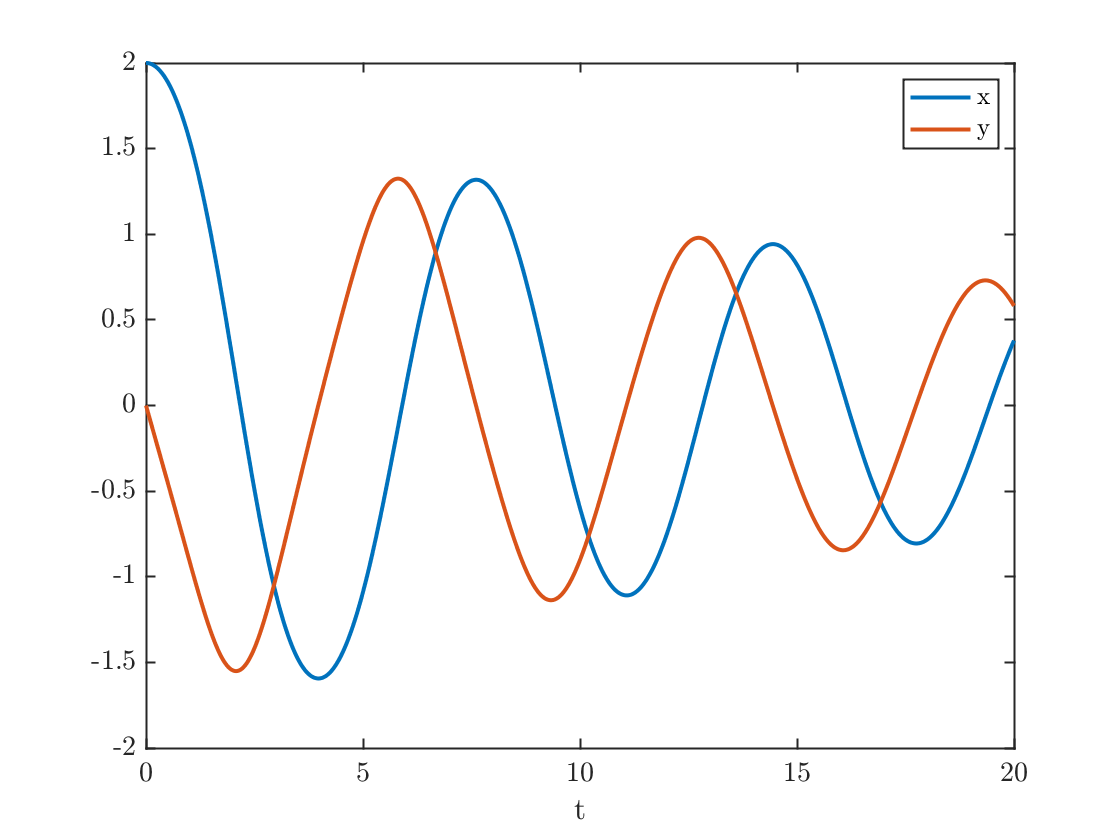## Plot the solution in the phase plane

plot(x,y)
xlabel('x');ylabel('y');